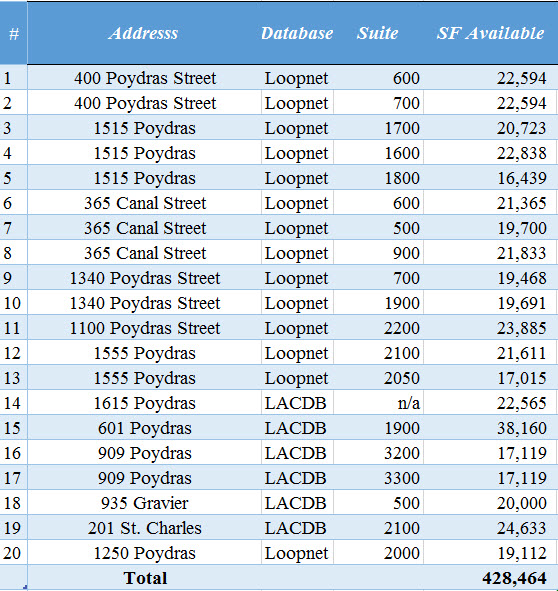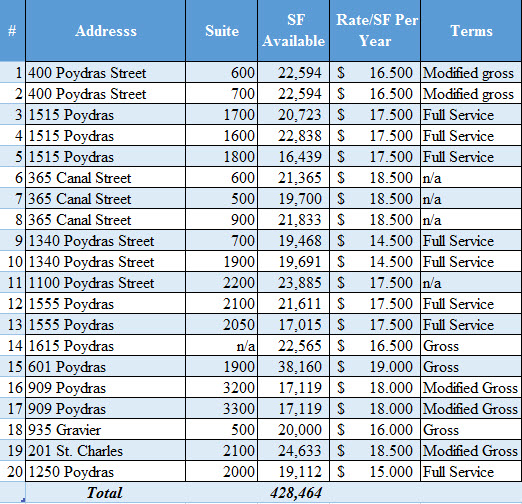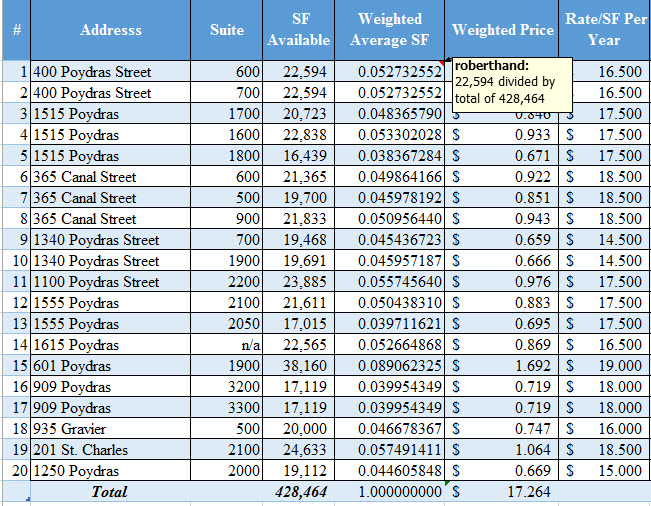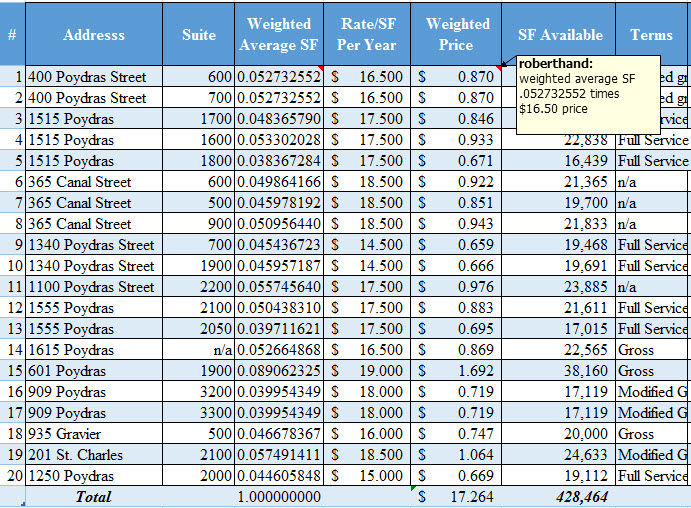### Jethro Bodine Ciphers New Orleans Class A Office Rates

Sharejethro bodine ciphering

Whenever Beverly Hillbillies star Jethro Bodine needed to do math, he would commence to ciphering by the cement pond, proving that math can be simple if you have enough fingers and toes. Commercial real estate is sometimes that way but sometimes not. Fortunately, when we need to do some math to answer the simple question, “What is the average price of a full floor of Class A Office space in New Orleans?”, the data is easily available and the work has already been done. There is just one catch: you can’t get the answer the way you were taught.

# Weighting The Average Is Not That Heavy

There are 14 Class A office buildings in New Orleans CBD, with a total square footage of approximately 9 million square feet, with one million square feet of that currently for lease, and 428,464 square feet of that comprised of full floor space.

## TABLE ONE: New Orleans Class A Full Floor Spacelist full floor space

Prices range from \$15 per square foot for sublease space to \$19 per square foot, and each space varies in how it passes along the cost of taxes, insurance and common area maintenance.

## TABLE TWO: List Rates of Class A Office Spaceprices full floor Class A

What is the average price? The simple way to determine the average price is to add up all the prices in the table above and divide by 20 ( the number of spaces), but that would not be the most reliable method because it assigns an equal weighting to each of the 20 spaces, as if they all had the same influence on the total square footage of 428,464. The best method for getting the average price is to take into account how much square footage each space has relative to the total. This way the number 15 space at 601 Poydras, which is the largest at 38,160 square feet, has a bigger impact on the final number than does the smallest space, number 5 at 1515 Poydras with only 16,439 square feet.

# There Are Only Two Intermediate Steps For The Weighted Average Number

Step 1 is to determine the percent each space is relative to the total. Take the square foot available and divide by the total (in space #1, 22,594 divided by 428,464). Space #1 amounts to about 5.27 percent of the total space. Fill down and you can see that space #15 amounts to 8.9 percent of the total space and, therefore, its price has more weight on the total market than does any other space. Note the total of the weighted square feet equals 1.

## TABLE THREE: Weighted Square Feetweighted price process

Step 2 multiplies the weighted square footage by the listed rate to determine the weighted price for that space. The value for each space is how much that space contributes to the average price, taking into account the amount of square feet contributed to the total square feet. The total of all 20 spaces is \$17.264, which is the most reliable answer to “What Is The Average Price?”.

## TABLE FOUR: Weighted Priceweighted price times SF

# Summary

The weighted average method utilized here can provide more reliable results for any sum of data. It works best when there is a large variation in the variables, such as square footage and price. The big surprise is that list prices of Class A office space in the New Orleans CBD are fairly close together, and further adjustments could be made for the variance in terms. While other amenities can affect price and are the judgment of the tenant or landlord, using the weighted average price in negotiations can be a good place to start.